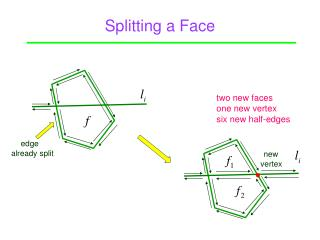# Splitting a Face - PowerPoint PPT PresentationDownload PresentationSplitting a Face

Splitting a Face
Download Presentation## Splitting a Face

- - - - - - - - - - - - - - - - - - - - - - - - - - - E N D - - - - - - - - - - - - - - - - - - - - - - - - - - -
##### Presentation Transcript

1. Splitting a Face Point two new faces one new vertex six new half-edges edge already split new vertex

2. The Algorithm • ConstructArrangement(L) • Compute a bounding box B(L) to contain all vertices of A(L)// • Initialize DCEL for B(L) // O(1) • fori←1 to n • doe ← edge on B(L) that first intersects with • f ← bounded face incident on e// O(i) • while f is bounded • split f • f ← next intersected face Point

3. Face Splitting Split faces in intersected by . Point A(L) not simple A(L) simple - Splitting a face f and finding next intersected face takes time linear in the complexity of f. - Insertion of takes time linear in total complexity of faces intersected by the line. - may leave f through a vertex v where ≥ 3 lines including intersect. f - Walk around v to find the next face (g) to split, scanning over edges that bound faces intersected by . g v

4. Zone Z(l) = { f | f is a face of A(L) intersected by l } Point four times once three times twice Complexity of Z = total complexity of faces (#vertices + #edges). A vertex may be counted up to four times.

5. Time of Arrangement Construction Zone Theorem Complexity of zone in an arrangement of m lines is O(m). Point ProofBy induction. Thus insertion of one line takes O(i) time. Time to insert all lines, and thus to construct line arrangement:

6. Solution to the Discrepancy Problem Point Continuous measure: Exactly one point  brute-force method At least two points  apply duality Discrete measure: Minimize h must have ≥ 1 points on its boundary.

7. How to Use Duality? A set S of n sample points Point A set S* of n lines A line through ≥ 2 sample points A vertex in arrangement A(S*) Becausep lies above l iff l* lies above p*,discrepancy problem reduces to the following. Problem 2For every vertex in A(S*), compute the numbers of lines above it, passing through it, and below it.

8. Reduction # lines above a vertex Point # lines below the vertex Sufficient to compute 2 of 3 numbers (with sum n). is known from DCEL. # lines through the vertex Need only compute the level of every vertex in A(S*).

9. Levels of Vertices in an Arrangement level of a point = # lines strictly above it. Point 0 1 1 2 2 2 3 3 3 4 3

10. Counting Levels of Vertices Point For each line l, compute the levels of vertices on it. intersections with n – 1 other lines Strategy: walk along l from left to right.

11. Counting Levels of Vertices no change of level between vertices 2 2 Point 3 1 1 A line crossing comes either from above or from below (relative to the current traversal position) Along the line the level changes only at a vertex . 0 0 0 1 1 level( ) determined in O(n) time by checking how many of the n–1 remaining lines are above . coming from above a) from above  level( ) = level( ) – 1 coming from below b) from below  level( ) = level( ) + 1

12. Running Times Point Levels of vertices along a line computable in time. Levels of all vertices in a line arrangement can be computed in time. Discrete measures computable in time.

13. Duality in Higher Dimensions point Point hyperplane hyperplane point

14. Inversion point point Point The point is lifted to the unit paraboloid.

15. Image of a Circle Image of a point on the circle C has z-coordinate Point plane is the intersection of the plane P with the unit paraboloid.

16. Inside/Outside  Below/Above Point lies inside C iff is below .